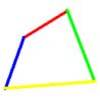#### You may also like### Areas and Ratios

Do you have enough information to work out the area of the shaded quadrilateral?### Biggest Bendy

Four rods are hinged at their ends to form a quadrilateral. How can you maximise its area?### So Big

One side of a triangle is divided into segments of length a and b by the inscribed circle, with radius r. Prove that the area is: abr(a+b)/ab-r^2

# Diagonals for Area

##### Age 16 to 18Challenge Level

Prove that the area of a quadrilateral is given by half the product of the lengths of the diagonals multiplied by the sine of the angle between the diagonals.